# The MODEL Procedure

### Tests on Parameters

In general, the hypothesis tested can be written as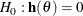where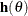is a vector-valued function of the parametersgiven by the r expressions specified on the TEST statement.

Let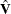be the estimate of the covariance matrix of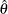. Letbe the unconstrained estimate ofand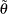be the constrained estimate ofsuch that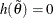. Let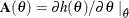Let r be the dimension of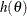and n be the number of observations. Using this notation, the test statistics for the three kinds of tests are computed as follows.

The Wald test statistic is defined as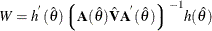The Wald test is not invariant to reparameterization of the model (Gregory and Veall 1985; Gallant 1987, p. 219). For more information about the theoretical properties of the Wald test, see Phillips and Park (1988).

The Lagrange multiplier test statistic is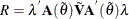where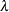is the vector of Lagrange multipliers from the computation of the restricted estimate.

The Lagrange multiplier test statistic is equivalent to Rao’s efficient score test statistic: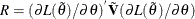where
is the log-likelihood function for the estimation method used. For SUR, 3SLS, GMM, and iterated versions of these methods, the likelihood function is computed as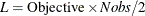For OLS and 2SLS, the Lagrange multiplier test statistic is computed as: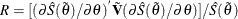where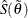is the corresponding objective function value at the constrained estimate.

The likelihood ratio test statistic is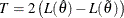whererepresents the constrained estimate ofandis the concentrated log-likelihood value.

For OLS and 2SLS, the likelihood ratio test statistic is computed as: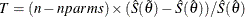This test statistic is an approximation from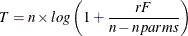when the value of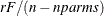is small (Greene, 2004, p. 421).

The likelihood ratio test is not appropriate for models with nonstationary serially correlated errors (Gallant, 1987, p. 139). The likelihood ratio test should not be used for dynamic systems, for systems with lagged dependent variables, or with the FIML estimation method unless certain conditions are met (see Gallant 1987, p. 479).

For each kind of test, under the null hypothesis the test statistic is asymptotically distributed as arandom variable with r degrees of freedom, where r is the number of expressions in the TEST statement. The p-values reported for the tests are computed from the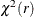distribution and are only asymptotically valid. When both RESTRICT and TEST statements are used in a PROC MODEL step, test statistics are computed by taking into account the constraints imposed by the RESTRICT statement.

Monte Carlo simulations suggest that the asymptotic distribution of the Wald test is a poorer approximation to its small sample distribution than the other two tests. However, the Wald test has the least computational cost, since it does not require computation of the constrained estimate.

The following is an example of using the TEST statement to perform a likelihood ratio test for a compound hypothesis.

   test a*exp(-k) = 1-k, d = 0 ,/ lr;


It is important to keep in mind that although individual t tests for each parameter are printed by default into the parameter estimates table, they are only asymptotically valid for nonlinear models. You should be cautious in drawing any inferences from these t tests for small samples.